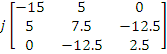# The matrix [A] = is decomposed into a product of a lower triangular matrix [L] and an upper triangular matrix [U]. The properly decomposed [L] and [U] matrices respectively are

1.   and

2.   and

3.   and

4.   and

4andExplanation :
No Explanation available for this question

# The two vectors [1,1,1] and [1,a,a2], where,are

1.  Orthonormal

2.  Orthogonal

3.  Parallel

4.   Collinear

4

Orthogonal

Explanation :
No Explanation available for this question

# A three -phase 440V, 6 pole, 50Hz, squirrel cage induction motor is running at a slip of 5%. The speed of stator magnetic field to rotor magnetic field and speed of rotor with respect to stator magnetic field are

1.  zero, - 5 rpm

2.  zero, 955 rpm

3.  1000rpm, -5rpm

4.  1000rpm, 955rpm

4

zero, - 5 rpm

Explanation :
No Explanation available for this question

# A capacitor is made with a polymeric dielectric having an εr of 2.26 and a dielectric breakdown strength of 50kV/cm. The permittivity of free space is 8.85pF/m. If the rectangular plates of the capacitor have a width of 20cm and a length of 40cm, then the maximum electric charge in the capacitor is

1.  2µC

2.  4µC

3.  8µC

4.  10µC

4

8µC

Explanation :
No Explanation available for this question

# The response h(t) of a linear time invariant system to an impulse δ( t), under initially relaxed condition is h(t) = e-t + e-2t. The response of this system for a unit step input u(t) is

1.  u(t) + e-t + e-2t

2.   (e-t + e-2t)u(t)

3.  (1.5 -e-t - 0.5e-2t)u(t)

4.  e-1δ( t) + e-2tu (t)

4

(1.5 -e-t - 0.5e-2t)u(t)

Explanation :
No Explanation available for this question

# The direct axis and quadrature axis reactance's of a salient pole alternator are 1.2p.u and 1.0p.u respectively. The armature resistance is negligible. If this alternator is delivering rated kVA at upf and at rated voltage then its power angle is

1.  300

2.  450

3.   600

4.   900

4

450

Explanation :
No Explanation available for this question

# A 4 ½ digit DMM has the error specification as : 0.2% of reading + 10 counts. If a dc voltage of 100V is read on its 200V full scale, the maximum error that can be expected in the reading is

1.  ±0.1%

2.  ±0.2%

3.  ±0.3%

4.  ±0.4%

4

±0.3\%

Explanation :
No Explanation available for this question

# A three - bus network is shown in the figure below indicating the p.u. impedances of each element The bus admittance matrix, Y-bus of the network is

1.

2.

3.

4.

4Explanation :
No Explanation available for this question

# A two loop position control system is shown below The gain k of the Tacho-generator influences mainly the

1.  Peak overshoot

2.  Natural frequency of oscillation

3.  Phase shift of the closed loop transfer function at very low frequencies (ω→0)

4.  Phase shift of the closed loop transfer function at very low frequencies (ω→α)

4

Peak overshoot

Explanation :
No Explanation available for this question

1.  00

2.  01

3.  10

4.  11

4